Select Page

# Part XX – C. The Axes of Creation

### 1776 and Spherical Time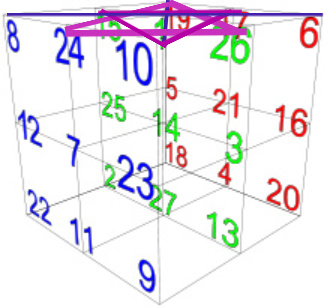In the previous section we made another connection with the year 1776 CE. Along that same axis through the vertical center of the Merkabah, a Star of David can be formed asymmetrical or opposite to the 3 parallel Stars and crosshairs that fill the 3 vertical tiers, as previously discussed. This new one is in the topmost tier, centered on the number 1, and its coordinates are (10-15-17) and (19-24-26) with the same diagonal crosshairs as before.  The total value for this new Star of David and its crosshairs is 1776. For anyone that has not been following this series from its inception, you can find the entire work here.

Now, we want to explain that the number and date 1776 is also found within the first 1000 digits of Pi. This was the year that the United States was founded (1776 CE) and in that same year, two months earlier, the illuminati was also founded. The exact digital sequence in Pi is …171776 and is found at digit number 888, exactly one half of 1776. For those that understand the 17 was purposely designed to precede 1776 and for those that do not, just know that the answers are found in Questioning everything.  Most telling is that the corresponding year in the 3760 sphere of time is –1984 and the ratio of those two corresponding years (1984/1776) is 1.117117117, while the ratio of 1776 to 3760 is 2.11711711…. I promise we are coming to the moment for explaining spherical time.  Meanwhile, the year 1776 HC in the corresponding Hebrew Calendar was also when the Tower of Babel took place and when G-d intervened to thwart the Erev Rav’s initial plan for a New World Order.  The year 1776 CE was their rebirth.  By the way, with the extra year in the actual counting from year 1, it is notable that the logarithm of 5778 is 3.7617, just like the Creator intended.

### Spiritual and Terrestrial Time

The dichotomy of Heaven and Earth built into the Torah’s very first verse, “אֵת הַשָּׁמַיִם, וְאֵת הָאָרֶץ” Heaven and Earth, lays out the two parallel spiritual and the physical time frames. It’s notable as we will see that the phrase “אֵת הַשָּׁמַיִם וְאֵת הָאָרֶץ” has a numerical value of 1500, when the kolel of One is added, just like the highest word value in the Torah, also of numerical value 1500. The ordinal value of the first word in the 4-word phrase, Et (את), is 23, like the first Triplet in the Torah (ברא), giving it the complete value of 424, that of Moshiach Ben David.

Then when adding the ordinal value, the complete numerical value of the entire phrase is 1657, the year the Biblical Flood began, counting from year 1.  Meanwhile, the complete gematria value with the kolel for the 7-letter phrase for Heaven “אֵת הַשָּׁמַיִם”, corresponding to the spiritual time frame, is 888. The other top tier Star of David in the vertical axis has one triangle (חפף) with 888 as its value and the other with 666, giving it an average of 777, nearly matching the complete gematria value for the 7-letter phrase for Earth “וְאֵת הָאָרֶץ”, corresponding to the terrestrial time frame, 776.  Meanwhile and the 7 Triplet sequence within the 72 Triplets that we recently discussed has a value of 888.    Of further comparative note is that the ratio of 888 to 1657, or Heaven to the finality of corruption and evil in the year of the flood, is the same as the ratio of 2018 to 3760, which is G-d willing the finality of corruption and evil and the elevation to the freedom of the Spiritual realm.  Please note that in spherical time, as will be explained soon, both 2018 and 3760 are the same point in time, like two expanding bubbles meeting in this current time frame.

### Triplet Magen Davids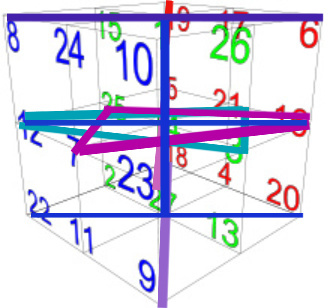In the prior two parts of this chapter, we have seen 3 Magen Davids complete with crosshairs lined up vertically through the 3 horizontal tiers of the Bird’s Nest Magical Cube of 42.  We also explained how the 27 ordinal values were systematically laid out in the cube by the Creator. They were laid out, vertical plane of 9 by vertical plane of 9, in 9 triplets (1,2,3; 4,5,6;…25,26,27) so that within each triplet no number would occupy the same plane as another, horizontal or vertical.  Well, that design resulted in the formation of intersecting triangles that formed yet another set of parallel Stars of David and the bars that bisect them.

This second set is perpendicular to the first set, and just like the first set its 36 total numbers sum to 6660. Also, like the first set, there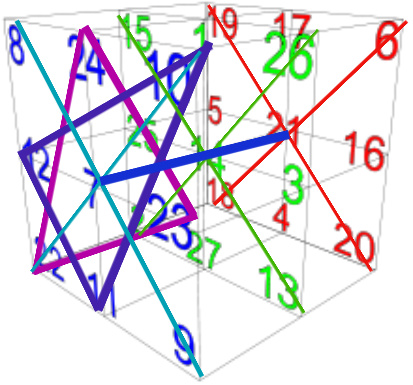is a ratio of 15 – 150 – 1500 built into the 3 planes of 9. In this case, one of the triangles in the front vertical plane (22,23,24 תךם) sums to 1500; a bar (13,14,15 מנס) in the middle plane sums to 150; and a triangle (4,5,6 דהו) in the rear plane sums to 15.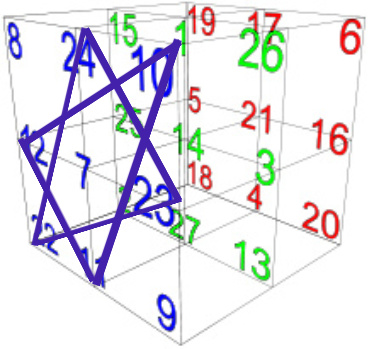Interestingly, the two crosshair bars of the front vertical plane add up to 441, the numerical value of Truth (emet), while the two triangles, (תךם) and (יכל) of the Star of David sum to 1560, or 10 x 156, the numerical value of Joseph and/or Zion. Now here is the kicker: the sum of the Star of David with its crosshairs in this vertical plane of 9 is (1560 + 441) = 2001, and the corner of the plane is 9 -11.

We should also point out that a separate (22 – 8 – 7 – 9 תחזט) triangle on this same vertical plane (face) equals 424, Moshiach Ben David.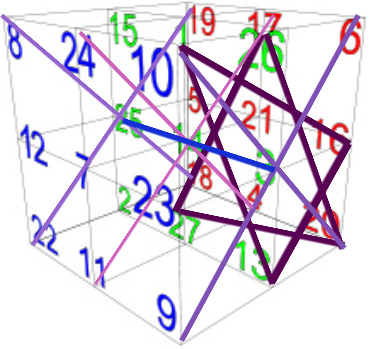There are, though, 3 axes to the cube, and as it turns out the 3rd axis also forms 3 parallel Stars of David with crosshairs that also sum to 6660. And just like the other two axes there is a ratio of 15 – 150 – 1500 built into its 3 tiers or planes as well.  Just like the second axis, one of the triangles in the front vertical plane (20,23,26 רךף) sums to 1500; a bar (11,14,17 כנפ) in the middle plane sums to 150; and a triangle (2,5,8 בהח) in the rear plane sums to 15.  The 3rd set and axis is perpendicular to the other 2 sets and axes.

While this progression of Yah (יה) from 1 to 10 to 100 in each of the 3 tiers in each of the 3 axes is of paramount importance in the cube’s Divine design, the 3 sums of (15 + 150 + 1500) total 4995, the sum of the 27 Hebrew letters. Please kind in mind, that the 27 letters used to compile these 3 sets are not the same as the Hebrew letters from 1 – 27, since some are duplicated, and some are not included at all. When we go from 1 to 10 to 100 we are expanding from Malchut to Zeir Anpin to Binah.  One of the Arizal’s Rosh Hashannah kavannot is to draw down the 150 Lights, specifically as 10 x Yah (יה).

If you recall, the vertical axis Stars were constructed entirely out of similar letter values, like 222 or 888.  When we examine the counter or opposite Stars in that same vertical axis, all 3 of them are wholly comprised of triangles that sum to either 15, 150, or 1500. The uppermost one has a triangle of 150, and another of 1500, and a bar of 15, and with the other bar of 111, it gave us 1776. The middle tier one has a triangle of 15, and another one of 1500, for a total of 1515. The bottommost tier has a triangle of 15, and another of 150, with a bar of 1500.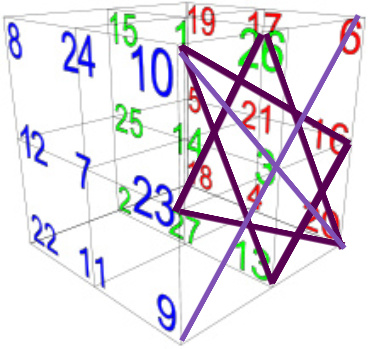We saw several interesting connections in the front face, or in other words the front plane, of the second axis (8-10-9-22), and find the same with the front face/plane of the third axis(10-6-20-9). The sum of the two triangles of the Star formed in this plane add up to (1500 + 120) = 1620, an obvious connection to Binah (1000) and Keter (620) and a less obvious connection of the 6 points of this Star to the 6 Matriarchs—Sarah, Leah, Rivka, Eve, Miriam, and Rachel whose collective complete value is 1620, as previously explained in conjunction with the 42-Letter Name matrix.  By the way, the Crosshair bars of that face equal 231, as in Abraham’s 231 Gates created by the 231 letter pair combinations of the Alef-bet.

In order to keep the Stars properly oriented and aligned throughout the 3 axes, we began each upper triangle in a specific corner. If we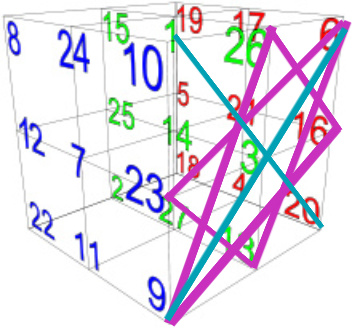were to create a different or opposite Star of that same third axis front face as the 1620 sum, by beginning its upper triangle with the NE corner instead of the NW one, the sum of its Star and crosshairs would be 1656, the Biblical date of the Flood.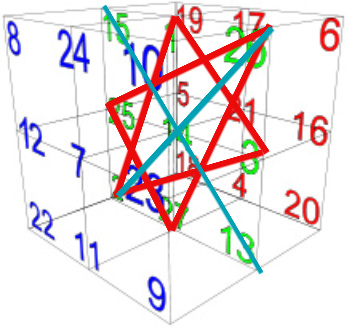Another date baked into the Magical Cube of 42 is found in the sum of the Star of David (2-1-3; 26-25-27) with its crosshairs (13-14-15; 2-14-26) in the middle of 2nd plane of the 2nd axis, which is 3408, as in the year 3408 HC, the year the 2nd Holy Temple was built.

It is also interesting that the sum of the crosshairs of the rear or third plane of the third axis is 1998 or (3 x 666).

Remember, this is a cube and as such it can be rotated along any of the 3-axes, so none are more than momentarily vertical or horizontal, and no face is any more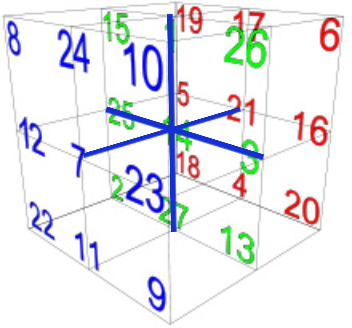considered the rear face than it is the front one.  All 3 sets of Stars of David with their crosshairs equal collectively (3 x 6660) or 19980, which also equals (4 x 4995), the sum total of the 27 letters in the Bird’s Nest. And in case you did not get the point in the previous section, 4995 = (45 x 111) and (3 x 6660) equals 19980 = (18 x 111 x 10) and the square root of 18 is 4.2426….

### Always 3

33 Letters, 3 Stars in each axis, 3 units in each Triplet, 3 Axes, 3 Planes in each axis, 3 Tiers in each and axis, 3 Patriarchs, and 3 columns in the Tree-of-Life, 3 visible dimensions to our existence, 3 aspects to time.

### Rotation on the Axes

With simultaneous rotation in the 3 axes the cube could be, like the electron, perpetually spinning unless we stop to observe it. This spinning cube or orb is spinning on a central point, the letter Nun (נ) and where do we find a Torah reference to this? In both Numbers 10:35-36 and Psalms 107 where we find an inverted Nun (נ), usually horizontally flipped, sometimes vertically, occasionally both, depending on who is interpreting it. This occurs with no other letter in the Torah or Tanakh. The two verses in the Numbers 10:35-36 represent an inversion of the Torah. They have 12 words and 7 words respectively, while the Torah first and last verses are 7 and 12 respectively. There are 85 letters in this verse and 58 words, verses, and letters in the Torah.  These two verses are about the Ark of the Covenant, when it goes and when it comes, and thanks to Ezra, we recite it 3 times a week when the Torah is removed from the Ark for reading. The Ark (הארן) has a numerical value of 256 or 28 and is the inverse of the Torah in that 58 = 390,625 and 1/28 = .00390625.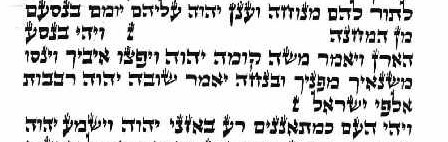It is said that this special window between the two inverted, or axially spun, Nuns (נ) belongs in another section, where the banners and locations of the 12 Tribes are to be placed, akin to the location of the Magical Cube with the 112 Triplets, just as two Nuns (נ) of 50 each and 12 equals 112. Others say that it represents a 7th Book of the Torah since it splits the Book of Numbers into 3 separate sections. Moreover, it is said that its 85 letters are representative of a much larger text.  Given the inversions we see demonstrated in the paragraph, we may guess that in comparison to the 58 words, verses, and letters in the Torah, there might be 85 words, verses, and letters in this hypothesized Book, and (58 + 85) = (424,000 – 607) = 424,000 – (500 + 107).

The 19 words, 2 verses, and 85 letters add up to 106, the numerical value of the spelled-out letter Nun(נון). With One for the Book or paragraph, it is 107.

It is in the 107th Psalm that we once again find the inverted Nuns (נ), and the 7 inverted Nuns (נ) therein begin sequentially with the 23rd verse, and as we know the 23rd Hebrew letter is Caf(ך) of numerical value 500. The number 424,000 as (424 x 1000), as Moshiach Ben David x Binah cannot be overlooked. What is this like? It is like the 107,007 letter in the Torah that begins the 10 Commandments.

Likewise, there are two places within the 72 Names, where back-to-back Triplets contain the letter Nun (נ), the first two are in the exact center of the Names, like the cube, at Triplet numbers 36 and 37, the same two that gave us the atomic numbers of Gold, Silver and Copper, and the other two are at Triplets 53 and 54 that sum to 107. With the kolel of 4, the 4 Triplets sum to 720, and with their ordinal location values, 900. The last and final Hebrew letter is Zadi (ץ) of numerical value 900 and when spelled out (צדי) with the kolel, it has a value of 107. The exact collective value of the first 3 Triplets is 256, the same as The Ark (הארן). The fourth Triplet connects with 107 in that (54 x 107) = 5778 and the 107th Triangular Number or alternatively, the sum of the integers from 1 – 107, is 5778.

There is nothing in the Torah that does not have deep meaning. There is nothing in the Torah that does not involve deep technology. There is nothing in the Torah that does not direct us towards obtaining and elevating to a state of Binah consciousness and the revelation of Moshiach Ben David.

How odd that the key to our solar system and universe is the number 273 and the key to our existence is the Cube of 27 Letters (Essential Forces) spinning in 3 axes!

Enjoy La’g B’Omer and connect to R’ Shimon Bar Yochai, author of the Zohar

Ezra